# R语言通过伽玛与对数正态分布假设下的广义线性模型对大额索赔进行评估预测

### 精算科学

``````> age=0:20
+ data=couts)
> Pgamma <- predict(reggamma.sp,newdata=data.frame(agevehi=age),type="response")``````

``````> plot(age,Pgamma,xlab="",ylab="",col="red",type="b",pch=4)
> lines(age,Pln,col="blue",type="b")``````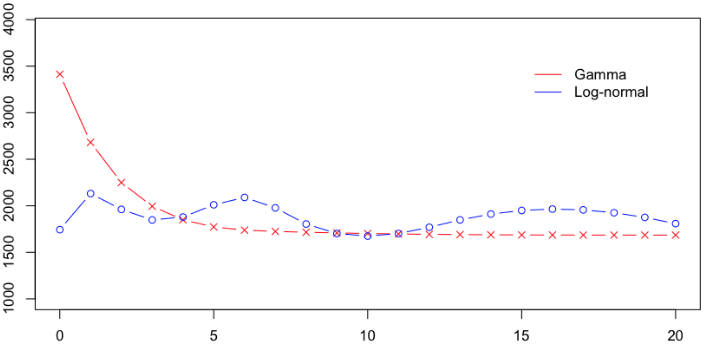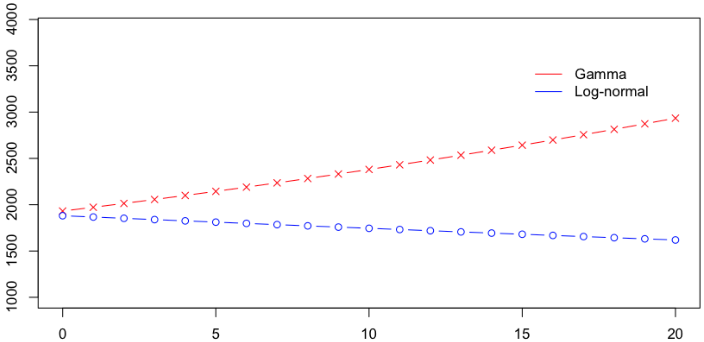``````> couts[which.max(couts\$cout),]
cout exposition zone puis agevehi ageconduct
7842  4024601       0.22    B         9          13            19
marque carbur densi region
7842      2         E      93     24``````

``````> s= 10000
> couts\$normal=(couts\$cout<=s)
> mean(couts\$normal)
 0.9818087``````

``````
> ypB=predict(regB,newdata=data.frame(agevehicule=age),type="response")
> ypB2=mean(couts\$cout[indice])``````

``````
> ypA=predict(regA,newdata=data.frame(agevehicule=age),type="response")
> ypA2=mean(couts\$cout[indice])``````

``````> ypC=predict(regC,newdata=data.frame(agevehicule=age),type="response")
> ypC2=predict(regC2,newdata=data.frame(agevehicule=age),type="response")``````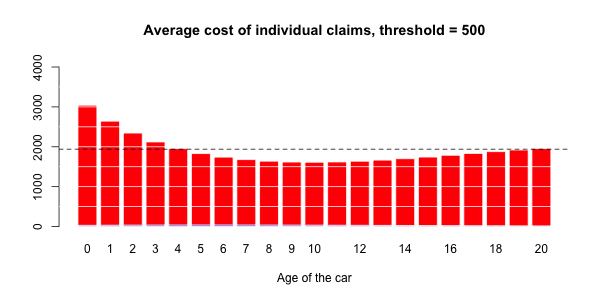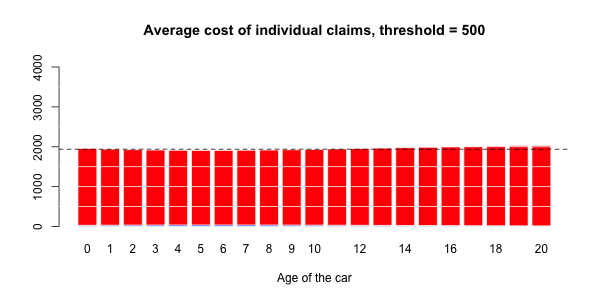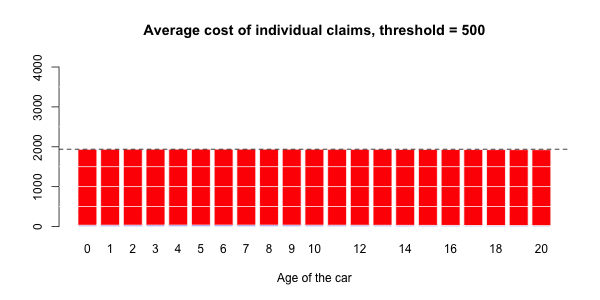Kaizong Ye拓端研究室（TRL）的研究员。

​非常感谢您阅读本文，如需帮助请联系我们！QQ在线咨询

15121130882

0571-63341498

## 关注有关新文章的微信公众号

This will close in 0 seconds